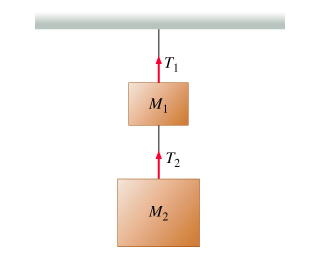# Problem: Two blocks with masses M1 and M2 hang one under the other. For this problem take the positive direction to be upward, and use g for the magnitude of the acceleration due to gravity1.  Find T2, the tension in the lower rope2. Find T1, the tension in the upper ropeFor question 3 and 4 the blocks are now acceleration upward due to the tension in the strings with acceleration of magnitude a.3. Find T2, the tension in the lower rope4. Find T1, the tension in the upper ropeExpress answers in terms of M1, M2 and g

###### FREE Expert Solution

Newton's second law:

$\overline{){\mathbf{F}}{\mathbf{=}}{\mathbf{m}}{\mathbf{a}}}$

(1)

ΣF = ma

T2 - M2g = 0

T2 = M2g

79% (226 ratings)###### Problem Details

Two blocks with masses M1 and M2 hang one under the other. For this problem take the positive direction to be upward, and use g for the magnitude of the acceleration due to gravity
1.  Find T2, the tension in the lower rope
2. Find T1, the tension in the upper rope

For question 3 and 4 the blocks are now acceleration upward due to the tension in the strings with acceleration of magnitude a.
3. Find T2, the tension in the lower rope
4. Find T1, the tension in the upper rope

Express answers in terms of M1, M2 and g